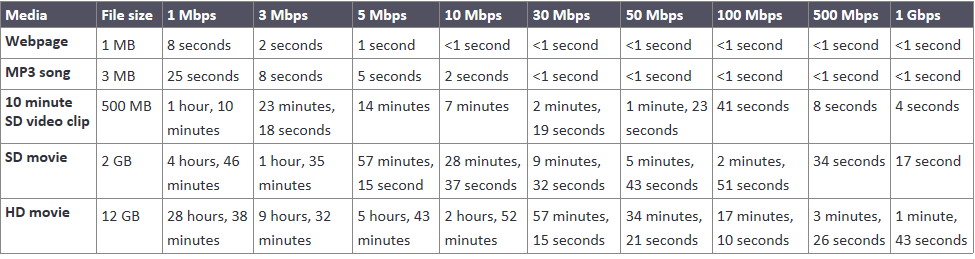# Bit vs Byte (Mbps vs MBps)

###### What is the difference between a bit and a byte?

A bit is the smallest unit of digital information, representing a binary value of either 0 or 1. On the other hand, a byte is a collection of 8 bits. It is commonly used to represent a single character or a small amount of data.

###### How do bits and bytes relate to internet speed?

Internet speed is typically measured in Mbps (MegaBits per second). This refers to the rate at which data can be transmitted over a network. One megabit equals one million bits.

When you download a file, the transfer rate is usually expressed in MBps (MegaBytes per second). Since a byte consists of 8 bits, the transfer rate in bytes will appear much lower compared to the speed in bits. This change in the unit is because the transfer rate is now measuring data volume rather than the transmission speed.

###### Why is the speed in Mbps faster than the speed in MBps?

As mentioned earlier, a byte contains 8 bits. Therefore, when you convert from Mbps to MBps, the numerical value decreases by a factor of 8. It may initially seem confusing, but it's important to understand the difference between transmission speed (bits) and data volume (bytes) when interpreting these values.

###### Which unit should I focus on when measuring internet speed?

When evaluating your internet speed, it is generally more relevant to focus on Mbps, as it directly represents the transmission rate. This measurement is commonly used by Internet service providers and network administrators. However, when downloading files or considering storage capacity, MBps becomes more significant as it represents the amount of data being transferred.

• Bit = 1
• Byte = 8 bits
• Megabit = 1,000,000 bits
• Megabyte 1,000,000 bytes
• Mbps = Mega Bits Per Second
• MBps = MegaBytes per second